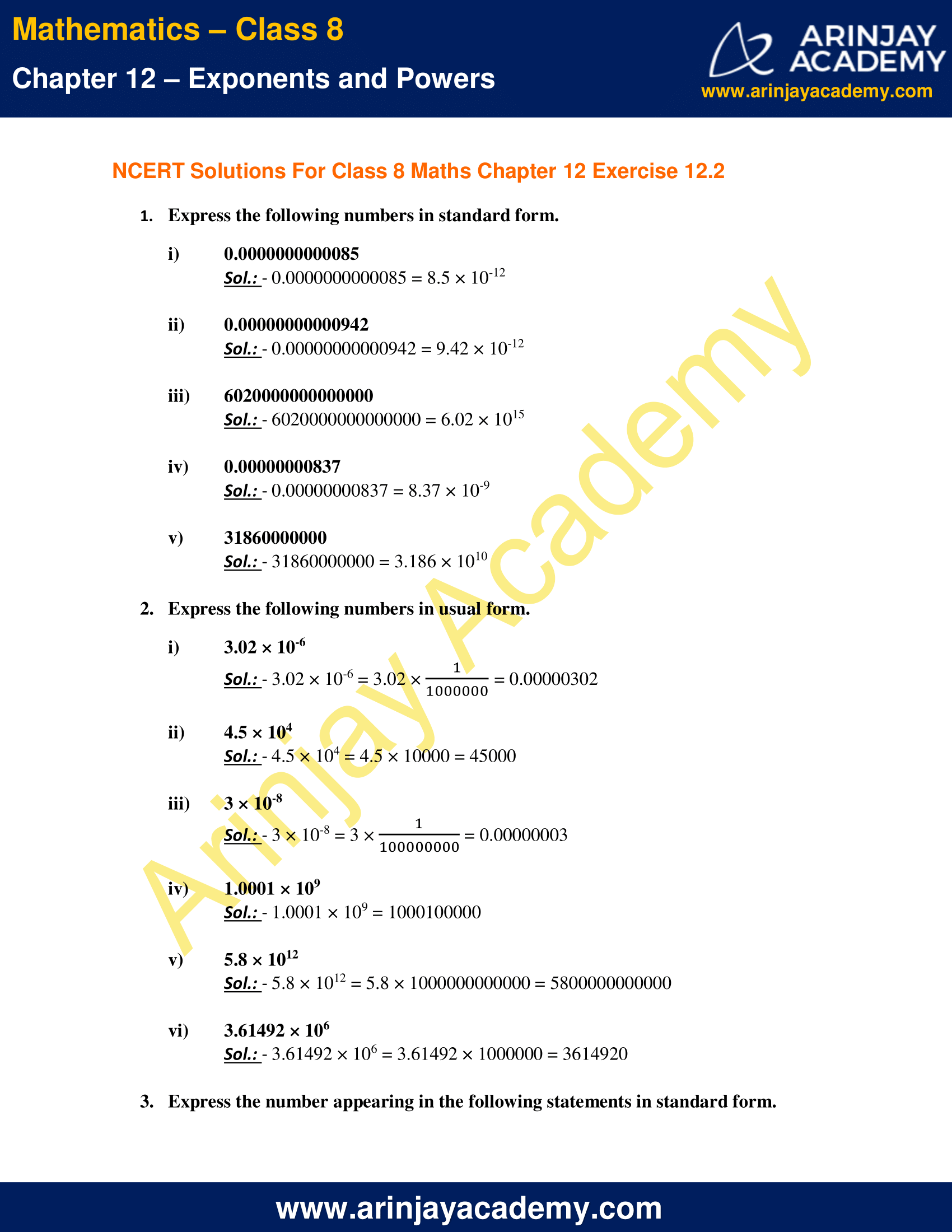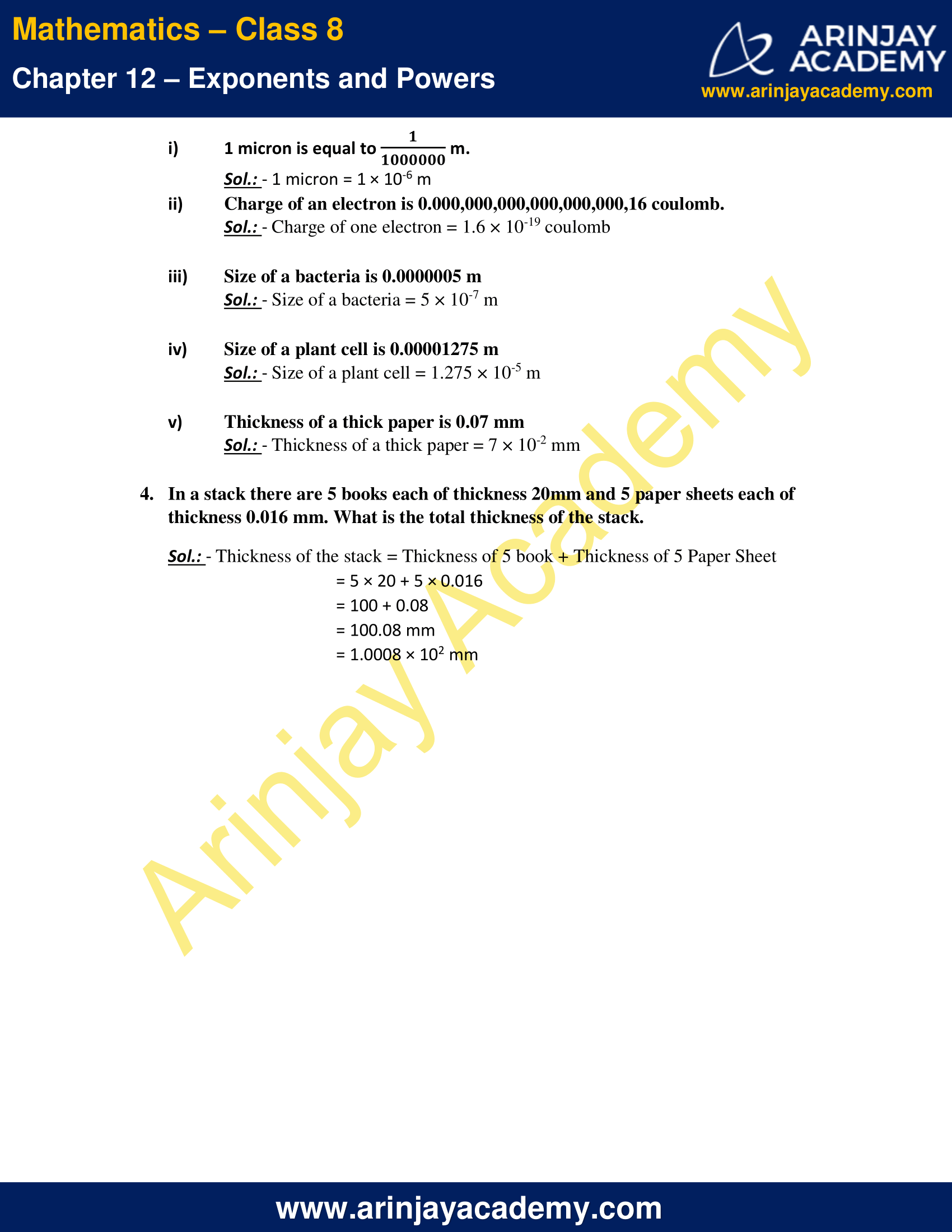# NCERT Solutions for Class 8 Maths Chapter 12 Exercise 12.2 – Exponents and Powers

NCERT Solutions for Class 8 Maths Chapter 12 Exercise 12.2 – Exponents and Powers, has been designed by the NCERT to test the knowledge of the student on the topic – Use of Exponents to Express Small Numbers in Standard Form – Comparing very large and very small numbers

### NCERT Solutions for Class 8 Maths Chapter 12 Exercise 12.2 – Exponents and PowersNCERT Solutions for Class 8 Maths Chapter 12 Exercise 12.2 – Exponents and Powers

1. Express the following numbers in standard form.

i) 0000000000085
Sol.: – 0.0000000000085 = 8.5 × 10-12

ii) 0.00000000000942
Sol.: – 0.00000000000942 = 9.42 × 10-12

iii) 6020000000000000
Sol.: – 6020000000000000 = 6.02 × 1015

iv) 00000000837
Sol.: – 0.00000000837 = 8.37 × 10-9

v) 31860000000
Sol.: – 31860000000 = 3.186 × 1010

2. Express the following numbers in usual form.

i. 3.02 × 10-6
Sol.: – 3.02 × 10-6 = 3.02 ×$\cfrac { 1 }{ 1000000 }$ = 0.00000302

ii. 4.5 × 104
Sol.: – 4.5 × 104 = 4.5 × 10000 = 45000

iii. 3 × 10-8
Sol.: – 3 × 10-8 = 3 ×$\cfrac { 1 }{ 100000000 }$  = 0.00000003

iv. 1.0001 × 109
Sol.: – 1.0001 × 109 = 1000100000

v. 5.8 × 1012
Sol.: – 5.8 × 1012 = 5.8 × 1000000000000 = 5800000000000

vi. 3.61492 × 106
Sol.: – 3.61492 × 106 = 3.61492 × 1000000 = 3614920

3. Express the number appearing in the following statements in standard form.

i) 1 micron is equal to$\cfrac { 1 }{ 1000000 }$.
Sol.: – 1 micron = 1 × 10-6 m

ii) Charge of an electron is 0.000,000,000,000,000,000,16 coulomb.
Sol.: – Charge of one electron = 1.6 × 10-19 coulomb

iii) Size of a bacteria is 0.0000005 m
Sol.: – Size of a bacteria = 5 × 10-7 m

iv) Size of a plant cell is 0.00001275 m
Sol.: – Size of a plant cell = 1.275 × 10-5 m

v) Thickness of a thick paper is 0.07 mm
Sol.: – Thickness of a thick paper = 7 × 10-2 mm

4. In a stack there are 5 books each of thickness 20mm and 5 paper sheets each of thickness 0.016 mm. What is the total thickness of the stack.

Sol.: – Thickness of the stack = Thickness of 5 book + Thickness of 5 Paper Sheet
= 5 × 20 + 5 × 0.016
= 100 + 0.08
= 100.08 mm
= 1.0008 × 102 mm

The next Exercise for NCERT Solutions for Class 8 Maths Chapter 13 Exercise 13.1 – Direct and Inverse Proportions can be accessed by clicking here

Download NCERT Solutions for Class 8 Maths Chapter 12 Exercise 12.2 – Exponents and Powers